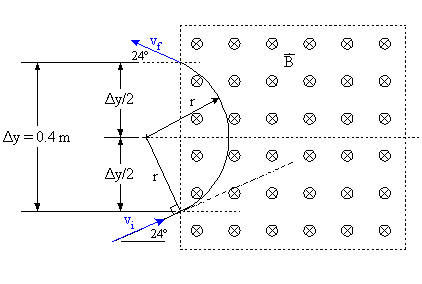# Finding v of a charged particle in a uniform magnetic field

• ThereIam

## Homework Statement

So there's a rectangular region in the xy plane subjected to a magnetic field B with strength 0.06T pointing in the -z. A proton moving in the xy plane enters the region with velocity v such that the velocity components are initially vx = v*cos(24), vy = v*sin(24). It leaves the rectangular region at a point 0.4m above (as in yf = yi + 0.4m, xf = xi) its entry with the same vy and opposite vx. What is v?

## Homework Equations

F = qv x B, qvB = mv^2/r

## The Attempt at a Solution

I figured I could just use F = qb x B to find the force in the y direction and then divided by the mass and integrated twice and set it equal to 0.4m, then solve for v, like

F/m = a = qvBcos(∅)/m
so=
x = 0.4 = ∫∫vqBcos(∅)/m d∅^2 + ∫vsin(θ)d∅ for ∅ = 24 to 154
then solve for v. The first integration is due to the B field and the second is just integrating the initial vy.

But I'm off by more than a couple orders of magnitude...

Any help would be appreciated! I suspect that my way isn't the easiest way, but if anyone could explain the flaw in my approach anyway that would be awesome.

Thanks for the help!

Last edited:
I think you can put away the heavy machinery if you draw the trajectory of the proton through the region and see if you can't locate the center of curvature (i.e., find the radius of curvature).

## Homework Equations

F = qv x B, qvB = mv^2/r

## The Attempt at a Solution

I figured I could just use F = qb x B to find the force in the y direction and then divided by the mass and integrated twice and set it equal to 0.4m, then solve for v, like

F/m = a = qvBcos(∅)/m
so=
x = 0.4 = ∫∫vqBcos(∅)/m d∅^2 + ∫vsin(θ)d∅ for ∅ = 24 to 154
then solve for v. The first integration is due to the B field and the second is just integrating the initial vy.

What is the direction of the force wit respect to the velocity of the proton? Does not it influence the x component of velocity ?

If you want to get the change of position by integrating acceleration, and then the velocity, you have to integrate with respect to what?

ehild

Just one more hint: In this case it helps to write out the equations of motion for $\vec{v}$ explicitly in components and then decouple them, leading to a 2nd order ODE for one component.

vanhees71, that's what I thought I had done in my attempt above. It should say "y = 0.4" But anyway vcos(∅) = vy. F in the y direction I thought equals vqBcos(∅)*m. I thought by breaking them into those components I had decoupled them. Could you elaborate? Thanks!

Last edited:
Once again I offer the suggestion that if you can determine the radius of curvature of the proton's trajectory while it is within the region of the magnetic field then you can determine the velocity of the proton without a lot of mathematical strife.

You are essentially told that the entry angle and exit angle are mirrored; on exit the horizontal velocity is the negative of that on entry, and the vertical velocity is the same as on entry. Further, you are given the separation of the entry and exit. You also know (or should know!) that the trajectory of a charged particle while in a magnetic field perpendicular to the plane of the motion must be circular in nature. Determining the radius of that circle is equivalent to determining the velocity (see the OP's Relevant Equations).

So I offer up the following diagram for consideration:#### Attachments

•1 person
Thank you very much for your responses gneill. I really do appreciate them, and I can see that they present a simpler approach to a solution.

I am still frustrated by my lack of understanding as to why my original approach didn't work. Something I'm trying to work on is my calculus skills (a great and unfortunate weakness of mine is in setting up problems appropriately using calculus) so even though your solution is both simpler and more elegant, I remain extremely curious where the problem in my original approach lay. Sorry that I did not express my gratitude for your thoughtful responses earlier.

I am still frustrated by my lack of understanding as to why my original approach didn't work. Something I'm trying to work on is my calculus skills (a great and unfortunate weakness of mine is in setting up problems appropriately using calculus) so even though your solution is both simpler and more elegant, I remain extremely curious where the problem in my original approach lay. Sorry that I did not express my gratitude for your thoughtful responses earlier.

You assumed that force acts only in the y direction. It is not true. The force is F=q[VxB], cross product of vectors V and B, and is perpendicular both to V and B. At the first instant, the force acts really in the y direction, and the particle accelerates and gains velocity in that direction. In the next instant, the direction of the velocity has both x and y component, and so is the acceleration. ax=(q/m)VyBz, and ay=-(q/m)VxBz.
You have to integrate the acceleration with respect time to get the velocity, and have to integrate velocity with respect time, to get displacement. You tried to integrate with respect theta. That was wrong.

ehild

•1 person